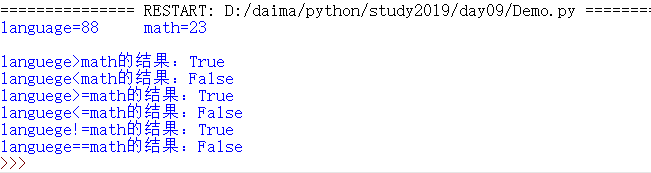• 相关内容（表格）
• 相关说明
• 源码
• 运行结果

> 大于
< 小于
== 等于
!= 不等于
>= 大于等于
<= 小于等于

## 源码

``````'''
# coding=utf-8
# 比较（关系）运算符
运算符    作用
>         大于
<         小于
==        等于
!=        不等于
>=        大于等于
<=        小于等于
'''

# 设语文为88分  数学为23分
language=88
math=23

# 用语文比较数学，用完每个比较运算符
# 标签提示，\t为空8格，\n为换行
print("language="+str(language)+"\tmath="+str(math)+"\n")
print("languege>math的结果："+str(language>math))       # 语文大于数学时
print("languege<math的结果："+str(language<math))		# 小于
print("languege>=math的结果："+str(language>=math))		# 大于等于
print("languege<=math的结果："+str(language<=math))		# 小于等于
print("languege!=math的结果："+str(language!=math))		# 不等于
print("languege==math的结果："+str(language==math))		# 等于
``````
• 1
• 2
• 3
• 4
• 5
• 6
• 7
• 8
• 9
• 10
• 11
• 12
• 13
• 14
• 15
• 16
• 17
• 18
• 19
• 20
• 21
• 22
• 23
• 24
• 25

## 运行结果